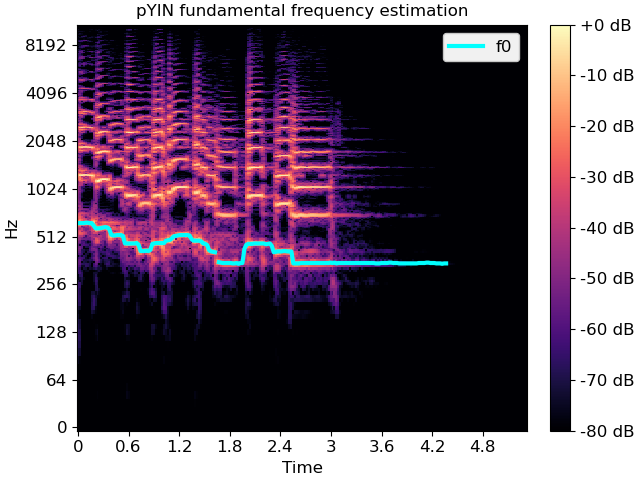# librosa.pyin¶

librosa.pyin(y, fmin, fmax, sr=22050, frame_length=2048, win_length=None, hop_length=None, n_thresholds=100, beta_parameters=2, 18, boltzmann_parameter=2, resolution=0.1, max_transition_rate=35.92, switch_prob=0.01, no_trough_prob=0.01, fill_na=nan, center=True, pad_mode='reflect')

Fundamental frequency (F0) estimation using probabilistic YIN (pYIN).

pYIN 1 is a modificatin of the YIN algorithm 2 for fundamental frequency (F0) estimation. In the first step of pYIN, F0 candidates and their probabilities are computed using the YIN algorithm. In the second step, Viterbi decoding is used to estimate the most likely F0 sequence and voicing flags.

1

Mauch, Matthias, and Simon Dixon. “pYIN: A fundamental frequency estimator using probabilistic threshold distributions.” 2014 IEEE International Conference on Acoustics, Speech and Signal Processing (ICASSP). IEEE, 2014.

2

De Cheveigné, Alain, and Hideki Kawahara. “YIN, a fundamental frequency estimator for speech and music.” The Journal of the Acoustical Society of America 111.4 (2002): 1917-1930.

Parameters
ynp.ndarray [shape=(n,)]

audio time series.

fmin: number > 0 [scalar]

minimum frequency in Hertz. The recomended minimum is librosa.note_to_hz('C2') (~65 Hz) though lower values may be feasible.

fmax: number > 0 [scalar]

maximum frequency in Hertz. The recomended maximum is librosa.note_to_hz('C7') (~2093 Hz) though higher values may be feasible.

srnumber > 0 [scalar]

sampling rate of y in Hertz.

frame_lengthint > 0 [scalar]

length of the frames in samples. By default, frame_length=2048 corresponds to a time scale of about 93 ms at a sampling rate of 22050 Hz.

win_lengthNone or int > 0 [scalar]

length of the window for calculating autocorrelation in samples. If None, defaults to frame_length // 2

hop_lengthNone or int > 0 [scalar]

number of audio samples between adjacent pYIN predictions. If None, defaults to frame_length // 4.

n_thresholdsint > 0 [scalar]

number of thresholds for peak estimation.

beta_parameterstuple

shape parameters for the beta distribution prior over thresholds.

boltzmann_parameter: number > 0 [scalar]

shape parameter for the Boltzmann distribution prior over troughs. Larger values will assign more mass to smaller periods.

resolutionfloat in (0, 1)

Resolution of the pitch bins. 0.01 corresponds to cents.

max_transition_ratefloat > 0

maximum pitch transition rate in octaves per second.

switch_probfloat in (0, 1)

probability of switching from voiced to unvoiced or vice versa.

no_trough_probfloat in (0, 1)

maximum probability to add to global minimum if no trough is below threshold.

fill_naNone, float, or np.nan

default value for unvoiced frames of f0. If None, the unvoiced frames will contain a best guess value.

centerboolean

If True, the signal y is padded so that frame D[:, t] is centered at y[t * hop_length]. If False, then D[:, t] begins at y[t * hop_length]. Defaults to True, which simplifies the alignment of D onto a time grid by means of librosa.core.frames_to_samples.

If center=True, this argument is passed to np.pad for padding the edges of the signal y. By default (pad_mode="reflect"), y is padded on both sides with its own reflection, mirrored around its first and last sample respectively. If center=False, this argument is ignored. .. see also:: np.pad

Returns
f0: np.ndarray [shape=(n_frames,)]

time series of fundamental frequencies in Hertz.

voiced_flag: np.ndarray [shape=(n_frames,)]

time series containing boolean flags indicating whether a frame is voiced or not.

voiced_prob: np.ndarray [shape=(n_frames,)]

time series containing the probability that a frame is voiced.

librosa.yin

Fundamental frequency (F0) estimation using the YIN algorithm.

Examples

Computing a fundamental frequency (F0) curve from an audio input

>>> y, sr = librosa.load(librosa.ex('trumpet'))
>>> f0, voiced_flag, voiced_probs = librosa.pyin(y, fmin=librosa.note_to_hz('C2'), fmax=librosa.note_to_hz('C7'))
>>> times = librosa.times_like(f0)


Overlay F0 over a spectrogram

>>> import matplotlib.pyplot as plt
>>> D = librosa.amplitude_to_db(np.abs(librosa.stft(y)), ref=np.max)
>>> fig, ax = plt.subplots()
>>> img = librosa.display.specshow(D, x_axis='time', y_axis='log', ax=ax)
>>> ax.set(title='pYIN fundamental frequency estimation')
>>> fig.colorbar(img, ax=ax, format="%+2.f dB")
>>> ax.plot(times, f0, label='f0', color='cyan', linewidth=3)
>>> ax.legend(loc='upper right')